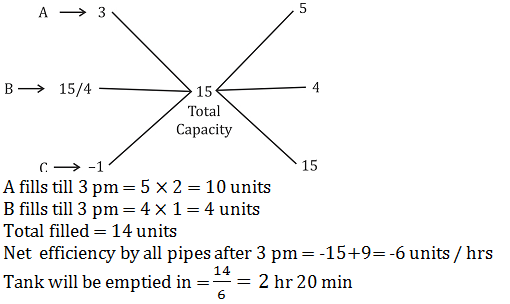Courses

# NABARD Quantitative Aptitude Practice: 5

## 30 Questions MCQ Test NABARD Assistant Manager Grade A Mock Test Series | NABARD Quantitative Aptitude Practice: 5

Description
This mock test of NABARD Quantitative Aptitude Practice: 5 for Banking Exams helps you for every Banking Exams entrance exam. This contains 30 Multiple Choice Questions for Banking Exams NABARD Quantitative Aptitude Practice: 5 (mcq) to study with solutions a complete question bank. The solved questions answers in this NABARD Quantitative Aptitude Practice: 5 quiz give you a good mix of easy questions and tough questions. Banking Exams students definitely take this NABARD Quantitative Aptitude Practice: 5 exercise for a better result in the exam. You can find other NABARD Quantitative Aptitude Practice: 5 extra questions, long questions & short questions for Banking Exams on EduRev as well by searching above.
QUESTION: 1

### Study the pie chart carefully to answer the questions that follow: Percentage breakup of number of children in five different villages and breakup of children attending school from those villages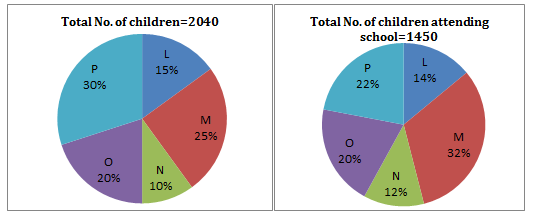Q. What is the respective ratio of total number of children from village O to the number of children attending school from the same village?

Solution: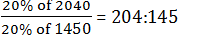QUESTION: 2

Solution:
QUESTION: 3

### Study the pie chart carefully to answer the questions that follow: Percentage breakup of number of children in five different villages and breakup of children attending school from those villagesQ. What is the total number of children not attending school from villages M and N together?

Solution:

No of children in village M and N together
= (25 + 10)% of 2040 = 714
No of children attending school from village M and N together
= (32 + 12)% of 1450 = 638.
Required No. of Children = 714 –638 = 76

QUESTION: 4

Study the pie chart carefully to answer the questions that follow:

Percentage breakup of number of children in five different villages and breakup of children attending school from those villagesQ. What is the total number of children from villages P and M together?

Solution:
QUESTION: 5

Study the pie chart carefully to answer the questions that follow:

Percentage breakup of number of children in five different villages and breakup of children attending school from those villagesQ. The number of children attending school from village L is approximately what percentage of the number of children from that village?

Solution: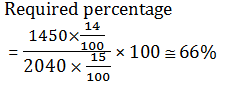QUESTION: 6

Study the table carefully to answer the questions that follow:

Number of people (in thousands) staying in 6 different cities and the percentage of men,women and children in those cities.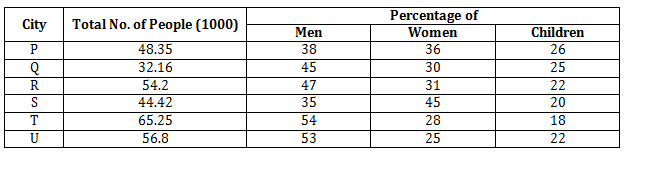Q. What is the ratio of the number of women from city R to that from city T?

Solution: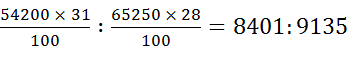QUESTION: 7

Study the table carefully to answer the questions that follow:

Number of people (in thousands) staying in 6 different cities and the percentage of men,women and children in those cities.Q. Which city has the lowest number of children?

Solution: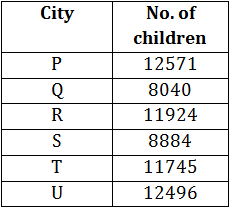QUESTION: 8

Study the table carefully to answer the questions that follow:

Number of people (in thousands) staying in 6 different cities and the percentage of men,women and children in those cities.Q. Total number of people from city U form approximately what per cent of the total number of people from all cities together?

Solution: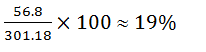QUESTION: 9

Study the table carefully to answer the questions that follow:

Number of people (in thousands) staying in 6 different cities and the percentage of men,women and children in those cities.Q. The number of women from city S forms what per cent of that from city P? (Rounded off to two digits after decimal)

Solution: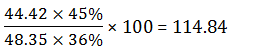QUESTION: 10

Study the table carefully to answer the questions that follow:
Number of people (in thousands) staying in 6 different cities and the percentage of men,women and children in those cities.Q. What is the average number of men from all the cities together?

Solution: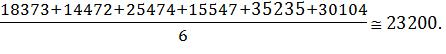QUESTION: 11

What will come in place of the question mark (?) in the following number series ?

3, 10, 32, 100, ?

Solution:

The pattern is : ×3 + 1, ×3 + 2, × 3 + 4, × 3 + 8

QUESTION: 12

What will come in place of the question mark (?) in the following number series ?

5, 3, 4, ?, 38

Solution:

The pattern is : × 1 – 2, × 2 – 2, × 3 –2, × 4 – 2

QUESTION: 13

What will come in place of the question mark (?) in the following number series ?

5, 6, ?, 57, 244

Solution: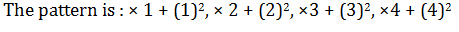QUESTION: 14

What will come in place of the question mark (?) in the following number series ?

3, 10, 21, ?, 51

Solution:

+7, +11, +13, +17.

QUESTION: 15

What will come in place of the question mark (?) in the following number series ?

5, 11, ?, 55, 117

Solution:

The pattern is : × 2 + 1, × 2 + 3, × 2 + 5, × 2 + 7

QUESTION: 16

Two equations (I) and (II) are given in each question. On the basis of these equations you have to decide the relation between ‘x’ and ‘y’ and give answer.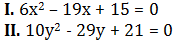Solution: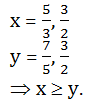QUESTION: 17

Two equations (I) and (II) are given in each question. On the basis of these equations you have to decide the relation between ‘x’ and ‘y’ and give answer.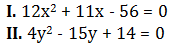Solution: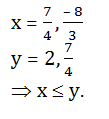QUESTION: 18

Two equations (I) and (II) are given in each question. On the basis of these equations you have to decide the relation between ‘x’ and ‘y’ and give answer.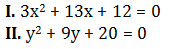Solution: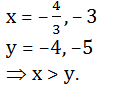QUESTION: 19

Two equations (I) and (II) are given in each question. On the basis of these equations you have to decide the relation between ‘x’ and ‘y’ and give answer.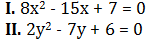Solution: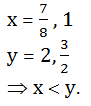QUESTION: 20

Two equations (I) and (II) are given in each question. On the basis of these equations you have to decide the relation between ‘x’ and ‘y’ and give answer.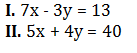Solution: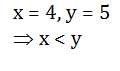QUESTION: 21

A house and a shop were sold for Rs. 1 lakh each, In this transaction, the house sale resulted into 20% loss whereas the shop sale into 20% profit. The entire transaction resulted in :

Solution: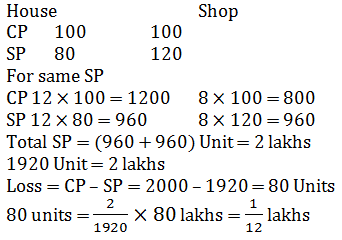QUESTION: 22

The marked price of an article is 50% above cost price. When marked price is increased by 20% and selling price is increased by 20%, the profit doubles. If original marked price is Rs. 300, then original selling price is

Solution: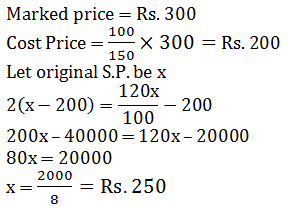QUESTION: 23

A boy aged 12 years is left with Rs. 100,000 which is under a trust. The trustees invest the money at 6% per annum and pay the minor boy a sum of Rs. 2500, for his pocket money at the end of each year. The expenses of trust come out to be Rs. 500 per annum. Find the amount that will be handed over to the minor boy after he attains the age of 18 years.

Solution: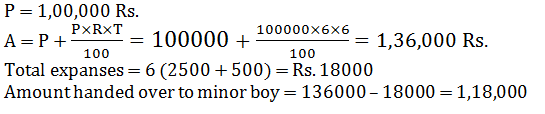QUESTION: 24

Sita deposited Rs. 5,000 at 10% simple interest for 2 years. How much more money will Sita have in her account at the end of two years. If it is compounded semiannually.

Solution: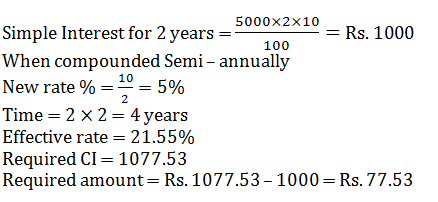QUESTION: 25

Three vessels whose capacities are in the ratio of 3 : 2 : 1 are completely filled with milk and water. The ratio of milk and water in the mixture of vessels are 5 : 2, 4 : 1 and 4 : 1 respectively. Taking 1/3 of first, ½ of second and 1/7 of third mixtures, a new mixture kept in a new vessel is prepared. The percentage of water in the new mixture is

Solution: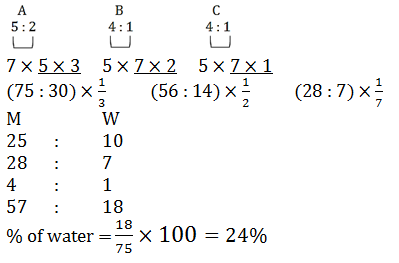QUESTION: 26

In an innings of a cricket match, three players A, B and C scored a total of 361 runs. If the ratio of the number of runs scored by A to that scored by B and also number of runs scored by B to that scored by C be 3 : 2, the number of runs scored by A was

Solution: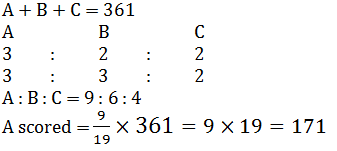QUESTION: 27

A container contains two liquids A and B in the ratio 7 : 5. When 9 litres of mixture are drawn off and the container is filled with B, the ratio of A and B becomes 1 : 1. How many litres of liquid A was in the container initially?

Solution: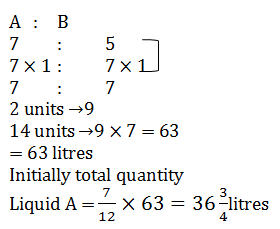QUESTION: 28

P can complete 1/4 of a work in 10 days, Q can complete 40% of the same work in 145 days. R, completes 1/3 of the work in 13 days and S can complete 1/6 of the work in 7 days. Who will be able to complete the work first?

Solution:
QUESTION: 29

A contractor undertakes to make a road in 40 days and employes 25 men. After 24 days, he finds that only one-third of the road is made. How many extra men should he employ so that he is able to complete the work 4 days earlier?

Solution: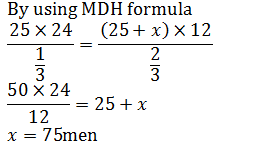QUESTION: 30

A water reservoir has two inlets and one outlet. Through the inlet it can be filled in 3 hours and 3 hours 45 minutes respectively. It can be emptied completely in 1 hour by the outlet. If the two inlets are opened at 01 : 00 pm and 02 : 00 pm respectively and the outlet at 03 : 00 pm then it will be emptied at.

Solution: